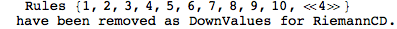# ToRiemann

 ToRiemann[expr] converts as many curvature tensors to Riemann tensors as possible. ToRiemann[expr, cd]only converts curvature tensors of the covariant derivative cd.
• ToRiemann converts the Weyl tensor, the symmetrized Riemann tensor, gradients of Christoffel symbols, the Kretschmann scalar, as well as the Riemann tensor with all indices down to Riemann tensors.
• If the curvature relations for the covariant derivative cd are not enabled, ToRiemann also converts curvature tensors of rank two to Riemann tensors.
Rank-two curvature tensors such as the Ricci tensor are only converted when the curvature relations are disabled:
 In:=
 Out=In:=
 Out=In:=
 Out=Out=Rank-two curvature tensors such as the Ricci tensor are only converted when the curvature relations are disabled:
 In:=Out=Out=In:=
 Out=In:=
 Out=In:=
 Out=The integrand of the Wald entropy formula,can be computed by varying a Lagrangianw.r.t. to the Riemann tensor. But because derived curvature tensors such as the Ricci and Weyl tensors don't explicitly depend on the Riemann tensor in xAct, we must convert them to Riemann tensors.
Let us take the square of the Weyl tensor as the Lagrangian:
And define a antisymmetric tensor for the binormal to the black hole horizon:
Convert the Lagrangian to Riemann tensors (note that curvature relations must be disabled for this to work, see ClearCurvatureRelations):
This can then be varied w.r.t. to the Riemann tensor by using VarD to obtain the integrand of the entropy:
Note that whenever your Lagrangian has covariant derivatives, you must first symmetrized them before taking the variation w.r.t. to the Riemann in order to get correct results for the entropy.